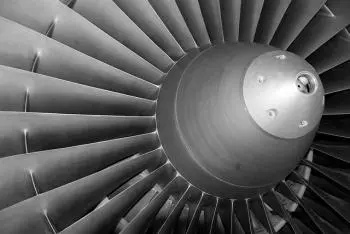# Angular Acceleration in KinematicsIn the field of kinematics, angular acceleration stands as a cornerstone in understanding the rotational motion of three-dimensional objects.

This concept, which is immersed in angular velocity and its derivatives with respect to time, displays a mathematical and conceptual complexity that enriches the appreciation of phenomena that range from the movement of the wheels of a vehicle to the contemplation of the stars in their cosmic rotation. .

The purpose of this article is to reveal the essential elements of angular acceleration, highlighting its mathematical formulation, its practical applications in engineering and astronomy, as well as its intricate connection with the moment of inertia and the law of conservation of angular momentum.

## What Is Angular Acceleration?

To understand angular acceleration, we must first become familiar with the concept of angular velocity. Angular velocity is the speed with which an object rotates around a point in a given time interval.

Angular acceleration, on the other hand, is the change in angular velocity as a function of time. In simpler terms, it is how quickly an object changes its rotation speed.

## Formulas and Units

Angular acceleration is measured in radians per second squared (rad/s²). To calculate it, we use the formula:Where

• α is the angular acceleration
• Δω is the change in angular velocity
• Δt is the change over time.

This formula allows us to quantify how the rotation speed of an object changes in a given period.

## Practical ApplicationsAngular acceleration is not just an abstract concept; It has practical applications in everyday life and in fields as diverse as engineering and astronomy.

For example, consider the wheels of a car. When we press the accelerator, we apply torque that induces angular acceleration in the wheels, making the car turn faster.

In astronomy, angular acceleration also plays a crucial role. Planets, stars and galaxies are in constant rotation.

## Moment of Inertia

To delve deeper into angular acceleration, we must mention the moment of inertia (I), which is the measure of the distribution of the mass of an object in relation to its axis of rotation. Moment of inertia is a key piece of the angular acceleration puzzle, as it determines how an object's mass affects its ability to change its rotational speed.

The relationship between moment of inertia, angular acceleration, and torque (the force that causes rotation) is encapsulated in the following formula:

τ = I · α

Where τ is the torque applied to the object.

This equation reveals the intimate connection between the distribution of an object's mass and its response to the forces that seek to make it spin.

## Conservation of Angular Momentum

Conservation of angular momentum is a law that states that if there is no external torque applied to a system, the total angular momentum of the system remains constant. In other words, the rotation of an object or system will not change unless an external torque is applied.

This law has astonishing consequences, from explaining a skateboarder's pirouette that reduces its moment of inertia to spin faster to the stability of satellites in orbit, where conservation of angular momentum guarantees predictable trajectories.

## Solved Example Exercises

Below are 2 examples of simple solved exercises on angular acceleration to understand the use of the formulas:

### Exercise 1: Calculation of Angular Acceleration

Suppose a disk rotates with an initial angular velocity of 3 rad/s and experiences an angular acceleration of 2 rad/s² for 4 s. We want to determine the final angular velocity of the disk.

Solution

Given the angular acceleration formula:Where wf is the final angular velocity and wi is the initial angular velocity, we can rearrange the formula to solve for the final angular velocity (ωf):

ω = ω i + α ⋅ Δt

We substitute the given values:

w = 3 rad/s + ( 2 rad/s² ⋅ 4 s )

Therefore, the final angular velocity of the disk is 11 rad/s

### Exercise 2: Moment of Inertia and Angular Acceleration

Suppose we have a solid cylinder of radius 0.5 m and mass 2 kg rolling on a non-slip surface with an angular acceleration of 4 rad/s². We want to determine the moment of inertia of the cylinder.

Solution

The moment of inertia (I) is related to the angular acceleration (α) and the torque (τ) by the formula:

τ = I · α

For a solid cylinder that rolls without slipping, the torque is equal to the product of the net force and the radius of the cylinder (τ=F⋅r). So, we can write:

F ⋅ r = I · α

We substitute the expression for the angular acceleration:

F ⋅ r = I ⋅ 4 rad/s²

The net force acting on the cylinder is simply the weight (mg). The radius (r) is 0.5 m. We substitute these values:

m ⋅ g ⋅ 0.5m = I ⋅ 4 rad/s²

We simplify and solve toWe substitute m=2 kg and g=9.8m/s²I=2.45kg⋅m²

Therefore, the moment of inertia of the cylinder is 2.45 kg⋅m²

Author:

Published: November 9, 2023
Last review: November 9, 2023